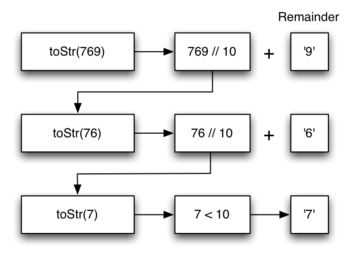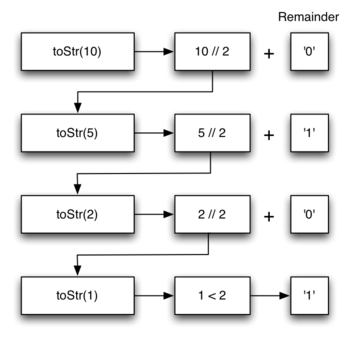# 16.4. Converting an Integer to a String in Any Base¶

Suppose you want to convert an integer to a string in some base between binary and hexadecimal. For example, convert the integer 10 to its string representation in decimal as "10", or to its string representation in binary as "1010". While there are many approaches one can take to solve this problem, the recursive formulation of the problem is very elegant.

Let’s look at a concrete example using base 10 and the number 769. Suppose we have a sequence of characters corresponding to the first 10 digits, like convString = "0123456789". It is easy to convert a number less than 10 to its string equivalent by looking it up in the sequence. For example, if the number is 9, then the string is convString or "9". If we can arrange to break up the number 769 into three single-digit numbers, 7, 6, and 9, then converting it to a string is simple. A number less than 10 sounds like a good base case.

Knowing what our base is suggests that the overall algorithm will involve three components:

1. Reduce the original number to a series of single-digit numbers.

2. Convert the single digit-number to a string using a lookup.

3. Concatenate the single-digit strings together to form the final result.

The next step is to figure out how to change state and make progress toward the base case. Since we are working with an integer, let’s consider what mathematical operations might reduce a number. The most likely candidates are division and subtraction. While subtraction might work, it is unclear what we should subtract from what. Integer division with remainders gives us a clear direction. Let’s look at what happens if we divide a number by the base we are trying to convert to.

Using integer division to divide 769 by 10, we get 76 with a remainder of 9. This gives us two good results. First, the remainder is a number less than our base that can be converted to a string immediately by lookup. Second, we get a number that is smaller than our original and moves us toward the base case of having a single number less than our base. Now our job is to convert 76 to its string representation. Again we will use integer division plus remainder to get results of 7 and 6 respectively. Finally, we have reduced the problem to converting 7, which we can do easily since it satisfies the base case condition of $$n < base$$, where $$base = 10$$. The series of operations we have just performed is illustrated in Figure 3. Notice that the numbers we want to remember are in the remainder boxes along the right side of the diagram.Figure 3: Converting an Integer to a String in Base 10¶

The activecode below shows the Python code that implements the algorithm outlined above for any base between 2 and 16.

Notice that in line 3 we check for the base case where n is less than the base we are converting to. When we detect the base case, we stop recursing and simply return the string from the convertString sequence. In line 6 we satisfy both the second and third laws–by making the recursive call and by reducing the problem size–using division.

Let’s trace the algorithm again; this time we will convert the number 10 to its base 2 string representation ("1010").Figure 4: Converting the Number 10 to its Base 2 String Representation¶

Figure 4 shows that we get the results we are looking for, but it looks like the digits are in the wrong order. The algorithm works correctly because we make the recursive call first on line 6, then we add the string representation of the remainder. If we reversed returning the convertString lookup and returning the toStr call, the resulting string would be backward! But by delaying the concatenation operation until after the recursive call has returned, we get the result in the proper order.

Self Check

Write a function that takes a string as a parameter and returns a new string that is the reverse of the old string.

Write a function that takes a string as a parameter and returns True if the string is a palindrome, False otherwise. Remember that a string is a palindrome if it is spelled the same both forward and backward. for example: radar is a palindrome. for bonus points palindromes can also be phrases, but you need to remove the spaces and punctuation before checking. for example: madam i’m adam is a palindrome. Other fun palindromes include:

• kayak

• aibohphobia

• Live not on evil

• Reviled did I live, said I, as evil I did deliver

• Go hang a salami; I’m a lasagna hog.

• Able was I ere I saw Elba

• Kanakanak – a town in Alaska

• Wassamassaw – a town in South Dakota